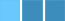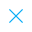Call Support +91-85588-96644

Keep me logged in
You can't leave Captcha Code empty
By submitting this form, you agree to the Terms & Privacy Policy.
ORTests given

OR# Statistics - Practice Exercise - 1

Questions

Time

Highest
score

Level

## ENGLISH

Language

Topics Covered: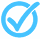StatisticsCalculating Mean of Grouped DataCalculating Mean of Grouped DataCalculating Mean of Grouped DataCalculating Mean of Grouped DataCalculating Mean of Grouped DataCalculating Mean of Grouped DataCalculating Mean of Grouped DataCalculating Mean of Grouped DataCalculating Mean of Grouped Data

### Latest Tests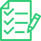#### NTSE Math - 6

30 Min - 20 Ques

Take Test#### NTSE Math - 5

30 Min - 20 Ques

Take Test#### NTSE Math - 4

30 Min - 20 Ques

Take Test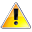This is a concept-building practice test and may not have exact structure as you would expect in the actual exam. Please exercise your discretion to attempt it or go to structured Featured Section.

Description:
Statistics - Practice Exercise - 1